# Introduction to Control Systems 16

16. Spring constant in force-voltage analogy is analogous to :

(a) Capacitance
(b) Reciprocal of capacitance
(c) Current
(d) Resistance

Explanation :

Analogous Quantities :

 Electrical Quantity Mechanical Analog I Mechanical Analog II (Force-Current) (Force-Voltage) Voltage, e Velocity, v Force, f Current, i Force, f Velocity, v Resistance, R Lubricity, 1/B (Inverse friction) Friction, B Capacitance, C Mass, M Compliance, 1/K (Inverse spring constant) Inductance, L Compliance, 1/K (Inverse spring constant) Mass, M Transformer, N1:N2 Lever, L1:L2 Lever, L1:L2

Force-Current Analogy :

1. Mass (M) is analogous to Capacitor (C).
2. Force is analogous to current I.
3. Displacement (x) is analogous to flux (ψ).
4. Coefficient of friction (B) is analogous to resistance 1/ R and
5. Spring constant K is analogous to inverse of the inductor (L).

Force-Voltage Analogy :

1. Mass (M) is analogous to inductance (L).
2. Force is analogous to voltage V.
3. Displacement (x) is analogous to charge (Q).
4. Coefficient of friction (B) is analogous to resistance R and
5. Spring constant is analogous to inverse of the capacitor (C).

General Knowledge Books##General Knowledge 2019 Price: Check on Amazon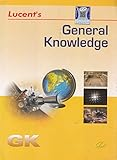-17% General Knowledge Price: INR 179.00 Was: INR 216.00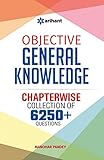-43% Objective General Knowledge Chapterwise Collection of 6250+ Questions Price: INR 169.00 Was: INR 295.00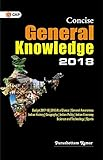Concise General Knowledge 2018 Price: INR 30.00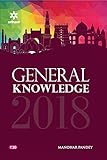General Knowledge 2018 (Old Edition) Price: Check on Amazon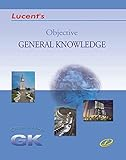-16% Objective General Knowledge Price: INR 302.00 Was: INR 360.00 ‹›

Be a Part of the New & Next

Share the Knowledge

Copy Protected by Chetan's WP-Copyprotect.